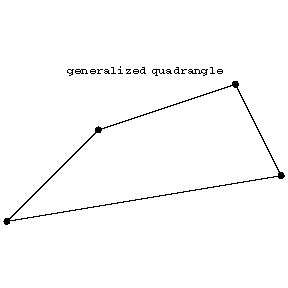##### Actions

In elementary geometry a quadrangle is a figure consisting of four segments intersecting in four (corner) points.Figure: q076000a

Note that each point is incident with 2 lines, each line is incident with 2 points, that there is at most one line passing through two distinct points, that two lines intersect in at most one point, and that for a point and a line not incident with that point there is a unique line through that point intersecting the given line.

These properties exemplify the simplest case of a generalized quadrangle. This is an incidence system $( P, B, I)$, i.e. a (symmetric) incidence relation $I \subset P \times B$ between points (the set $P$) and lines (or blocks, the set $B$) such that

i) for each point $M$ and line $p$ not passing through $M$ there is precisely one pair $( N, n)$ with $M$ on $n$, $N$ on $n$ and $p$.

A generalized quadrangle can be seen as a very special kind of bipartite graph (cf. Graph, bipartite), obtained by taking as its vertex set the disjoint union $P\amalg B$ and with $M \in P$, $m \in B$ connected if and only if $M$ is on $m$.

Interchanging $P$ and $B$ one obtains the dual generalized quadrangle.

A generalized quadrangle is non-degenerate if there is no point that is collinear with all others, where two points are collinear if they are on a common line.

A finite generalized quadrangle of order $( s, t)$ is one that satisfies i) above and also

ii) each point is incident with precisely $t + 1$ lines and there is at most one line through two distinct points;

iii) each line has $s+ 1$ points and two lines intersect in at most one point.

A simple example of a finite generalized quadrangle of order $( 1, 2)$ is depicted belowFigure: q076000b

This is also an example of a grid, which is an incidence structure $( P, B, I )$ with $P = \{ {x _ {ij} } : {i= 1 \dots s _ {1} , j = 1 \dots s _ {2} } \}$, $B = \{ l _ {1} \dots l _ {s _ {1} } ; m _ {1} \dots m _ {s _ {2} } \}$ with $x _ {ij}$ on $l _ {k}$ if and only if $i = k$ and $x _ {ij}$ on $m _ {k}$ if and only if $j = k$.

There are three known families of generalized quadrangles associated with the classical groups; these are known as classical generalized quadrangles. There are also others, for instance coming from ovoids (cf. Ovoid).

Generalized quadrangles were introduced by J. Tits [a1] and he also described the classical ones and the first non-classical ones. More generally one considers generalized $m$- gons, [a4].

How to Cite This Entry: